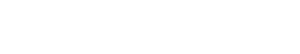# Arithmetic

Arithmetic is defined as a branch of mathematics that deals with the study of numbers, their operations, and their properties. Arithmetic is often used in conjunction with other mathematical disciplines such as geometry, algebra, and trigonometry.

Arithmetic can be performed on both whole numbers and fractions. The most basic arithmetic operations include addition, subtraction, multiplication, and division. More complex operations include exponentiation and roots. Arithmetic can also be applied to variables and functions.

## Using Arithmetic in Logistic Regression

Arithmetic is also used in the field of logistic regression. This technique is used to model the relationship between a dependent variable and one or more independent variables. The dependent variable is usually binary, which means it can take on two values: 0 or 1. The independent variables can be anything from continuous (like age) to categorical (like gender). Logistic regression can be used to predict the probability of an event occurring. For example, if we wanted to predict whether or not a student would pass their exams, we could use logistic regression to build a model that takes into account factors such as the student’s age, their previous grades, and the difficulty of the exam.

## Using Arithmetic for Machine Learning

In the field of machine learning, arithmetic is used to define the relationships between data points. These relationships can be linear or nonlinear. Linear relationships are those that can be represented by a straight line, while nonlinear relationships are more complex and cannot be represented by a straight line. In machine learning, we often use linear models to represent data so that we can make predictions about new data points. For example, if we have a dataset of students’ ages and heights, we can use linear regression to find the relationship between these two variables. Then, we can use this model to predict the height of a new student based on their age.

## Using Arithmetic for Word Segmentation

Arithmetic is also used in the field of word segmentation. This is the process of dividing a string of text into its individual words. In order to do this, we need to be able to identify where one word ends and the next begins. This can be difficult, especially in languages that don’t use spaces between words (like Chinese). One way to segment words is by using a statistical model called a Hidden Markov Model (HMM). This model can be trained to identify the boundaries between words. Once the model is trained, it can then be used to segment new strings of text.Unlock the power of actionable insights with AI-based natural language processing.